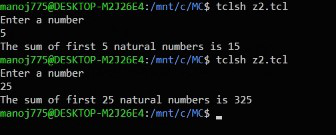# TCL script to find sum of n natural numbers using looping statements

• Last Updated : 26 Apr, 2021

In this article, we will discuss the overview of TCL script and will cover the TCL script to find the sum of n natural numbers using looping statements with the help of an example. Let’s discuss it one by one.

Pre-requisite –

Overview :
We will understand the syntax of the while loop and for loop in Tool Command Language with a simple example. In this example, we will first use the while loop to find the sum of the first n natural numbers, and then we will see how to use for loop to accomplish the same. We will also compare the syntax with a familiar language to understand it better.

While-loop implementation :
We will discuss the implementation steps as follows.

Step-1 :
The first step is to read a number from the user after prompting to do so. To read the number we use gets, and we use puts to give a prompt.

```puts "Enter a number"
gets stdin b```

Step-2 :
Our next step is to initialize the sum to 0 and the iteration variable, i to 0. After this, we can have the while loop implementation of the code.

```set sum 0
set i 0
while {\$i<=\$b} {
set sum [expr \$sum+\$i]
incr i
}```

Note –
The syntax of the while loop must be exactly as shown above. If you neglect the spaces or type the opening curly brace in a new line, the result will be an error.

Step-3 :
The implementation of the while loop specified above would like the following in C programming as follows.

```sum=0;
i=0;
while(i<=b)
{
sum=sum+i;
i++;
}```

Step-4 :
Finally, the whole code and output are as follows.
Code –

```puts "Enter a number"
gets stdin b
set sum 0
set i 0
while {\$i<=\$b} {
set sum [expr \$sum+\$i]
incr i
}
puts "The sum of first \$b natural numbers is \$sum"```

Output :For-loop implementation :
We will discuss the implementation steps as follows.

Step-1 :
The first 2 lines of code are the same as in the while loop. So, let’s look at the for loop implementation part.

```set sum 0
for {set i 1} {\$i<=\$b} {incr i} {
set sum [expr \$sum+\$i]
}```

Note –
The syntax of for loop must be exactly as shown above. If you neglect the spaces or type the opening curly brace in a new line, the result will be an error.

Step-2 :
The implementation of the for loop specified above would like the following in C programming as follows.

```sum=0;
for(i=1;i<=n;i++)
{
sum=sum+i;
}```

Step-3 :
Finally, let’s view the entire code and its output as follows.
Code –

```puts "Enter a number"
gets stdin b
set sum 0
for {set i 1} {\$i<=\$b} {incr i} {
set sum [expr \$sum+\$i]
}
puts "The sum of first \$b natural numbers is \$sum"```

Output :My Personal Notes arrow_drop_up